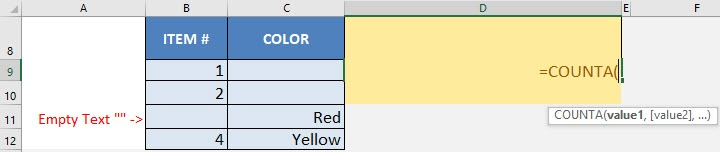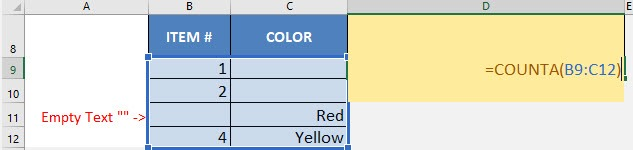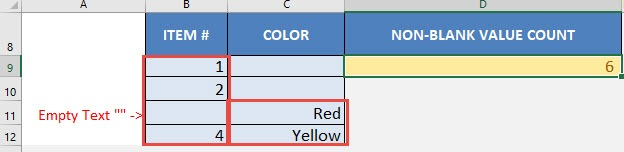What does it do?

Counts the number of cells that are non-blank/non-empty (including empty text “”)

Formula breakdown:

=COUNTA(value1, [value2], …)

What it means:

=COUNTA(value or range of cells to check, [value or range of cells to check], …)

Do you have a scenario where you want to count the number of cells that are non-blank or not empty?

I’m sure you do!  There is a simple way to count this with Excel’s COUNTA formula!

This formula counts everything: numbers, text, non-empty text “”, you name it!

I explain how you can do this below:

STEP 1: We need to enter the COUNTA function in a blank cell. Notice there are 6 non-blank cells in here:

## =COUNTA(STEP 2: The COUNTA arguments:

## value

What is the value or range of values that you want to check how many are non-blank?

## =COUNTA(B9:C12)You now have your count of values that are non-blank! There are 6 non-blank values!CountA Formula in Excel